# Distance Calculator

Created by Álvaro Díez
Reviewed by Dominik Czernia, PhD candidate and Jack Bowater
Last updated: May 04, 2022

Have you ever wanted to calculate the distance from one point to another, or the distance between cities? Have you ever wondered what the distance definition is? We have all these answers and more, including a detailed explanation of how to calculate the distance between any two objects in 2D space. As a bonus, we have a fascinating topic on how we perceive distances (for example as a percentage difference); we're sure you'll love it!

Prefer watching over reading? Learn all you need in 90 seconds with this video we made for you:

## What is distance?

Before we get into how to calculate distances, we should probably clarify what a distance is. The most common meaning is the /1D space between two points. This definition is one way to say what almost all of us think of distance intuitively, but it is not the only way we could talk about distance. You will see in the following sections how the concept of distance can be extended beyond length, in more than one sense that is the breakthrough behind Einstein's theory of relativity.

If we stick with the geometrical definition of distance we still have to define what kind of space we are working in. In most cases, you're probably talking about three dimensions or less, since that's all we can imagine without our brains exploding. For this calculator, we focus only on the 2D distance (with the 1D included as a special case). If you are looking for the 3D distance between 2 points we encourage you to use our 3D distance calculator made specifically for that purpose.

To find the distance between two points, the first thing you need is two points, obviously. These points are described by their coordinates in space. For each point in 2D space, we need two coordinates that are unique to that point. If you wish to find the distance between two points in 1D space you can still use this calculator by simply setting one of the coordinates to be the same for both points. Since this is a very special case, from now on we will talk only about distance in two dimensions.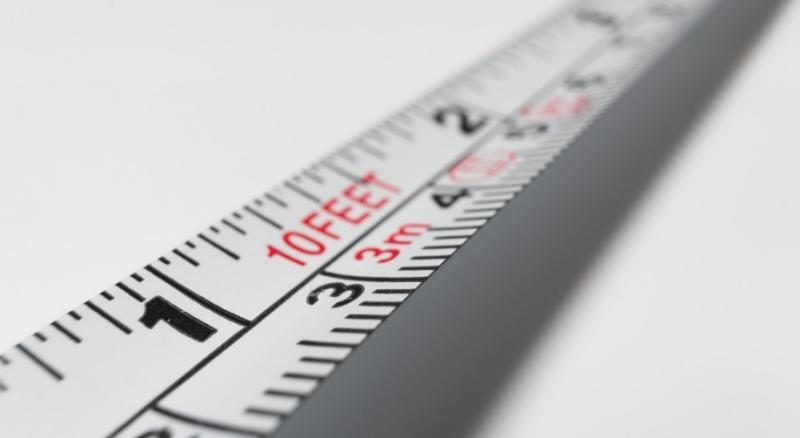The next step, if you want to be mathematical, accurate, and precise, is to define the type of space you're working in. No, wait, don't run away! It is easier than you think. If you don't know what space you're working in or if you didn't even know there is more than one type of space, you're most likely working in Euclidean space. Since this is the "default" space in which we do almost every geometrical operation, and it's the one we have set for the calculator to operate on. Let's dive a bit deeper into Euclidean space, what is it, what properties does it have and why is it so important?

## The distance formula for Euclidean distance

The Euclidean space or Euclidean geometry is what we all usually think of 2D space is before we receive any deep mathematical training in any of these aspects. In Euclidean space, the sum of the angles of a triangle equals 180º and squares have all their angles equal to 90º; always. This is something we all take for granted, but this is not true in all spaces. Let's also not confuse Euclidean space with multidimensional spaces. Euclidean space can have as many dimensions as you want, as long as there is a finite number of them, and they still obey Euclidean rules.

We do not want to bore you with mathematical definitions of what is a space and what makes the Euclidean space unique, since that would be too complicated to explain in a simple distance calculator. However, we can try to give you some examples of other spaces that are commonly used and that might help you understand why Euclidean space is not the only space. Also, you will hopefully understand why we are not going to bother calculating distances in other spaces.The first example we present to you is a bit obscure, but we hope you can excuse us, as we're physicists, for starting with this very important type of space: Minkowski space. The reason we've selected this is because it's very common in physics, in particular it is used in relativity theory, general relativity and even in relativistic quantum field theory. This space is very similar to Euclidean space, but differs from it in a very crucial feature: the addition of the dot product, also called the inner product (not to be confused with the cross product).

Both the Euclidean and Minkowski space are what mathematicians call flat space. This means that space itself has flat properties; for example, the shortest distance between any two points is always a straight line between them (check the linear interpolation calculator). There are, however, other types of mathematical spaces called curved spaces in which space is intrinsically curved and the shortest distance between two points is no a straight line.

This curved space is hard to imagine in 3D, but for 2D we can imagine that instead of having a flat plane area, we have a 2D space, for example, curved in the shape of the surface of a sphere. In this case, very strange things happen. The shortest distance from one point to another is not a straight line, because any line in this space is curved due to the intrinsic curvature of the space. Another very strange feature of this space is that some parallel lines do actually meet at some point. You can try to understand it by thinking of the so-called lines of longitude that divide the Earth into many time zones and cross each other at the poles.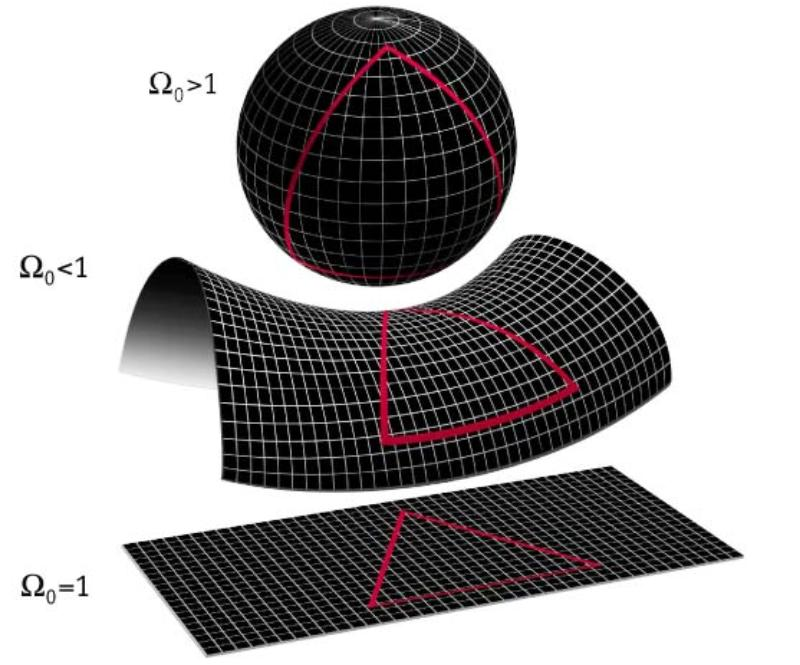It is important to note that this is conceptually VERY different from a change of coordinates. When we take the standard x, y, z coordinates and convert into polar, cylindrical, or even spherical coordinates, but we will still be in Euclidean space. When we talk about curved space we are talking about a very different space in terms of its intrinsic properties. In spherical coordinates, you can still have a straight line and distance is still measured in a straight line, even if that would be very hard to express in numbers.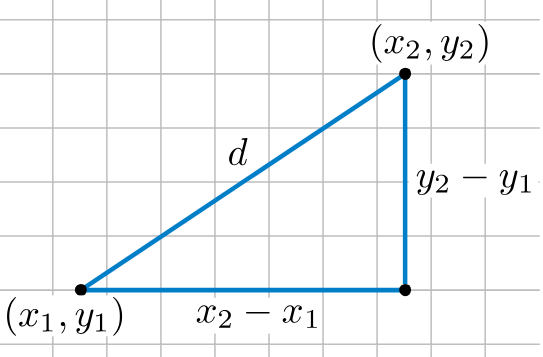Coming back to the Euclidean space, we can now present you with the distance formula that we promised at the beginning. The distance formula is

√[(x₂ - x₁)² + (y₂ - y₁)²],

which relates to the Pythagorean theorem: a² + b² = c². Here, a and b are legs of a right triangle and c is the hypotenuse. Suppose that two points, (x₁, y₁) and (x₂, y₂), are coordinates of the endpoints of the hypotenuse. Then (x₂ - x₁)² in the distance equation corresponds to a² and (y₂ - y₁)²corresponds to b². Since c = √(a² + b²), you can see why this is just an extension of the Pythagorean theorem.

An extended application of the distance between points can be found in the segment addition postulate, which involves finding a segment length when 3 points are collinear.

## Distance to any continuous structure

The distance formula we have just seen is the standard Euclidean distance formula, but if you think about it, it can seem a bit limited. We often don't want to find just the distance between two points. Sometimes we want to calculate the distance from a point to a line or to a circle. In these cases, we first need to define what point on this line or circumference we will use for the distance calculation, and then use the distance formula that we have seen just above.

Here is when the concept of perpendicular line becomes crucial. The distance between a point and a continuous object is defined via perpendicularity. From a geometrical point of view, the first step to measure the distance from one point to another, is to create a straight line between both points, and then measure the length of that segment. When we measure the distance from a point to a line, the question becomes "Which of the many possible lines should I draw?". In this case the answer is: the line from the point that is perpendicular to the first line. This distance will be zero in the case in which the point is a part of the line. For these 1D cases, we can only consider the distance between points, since the line represents the whole 1D space.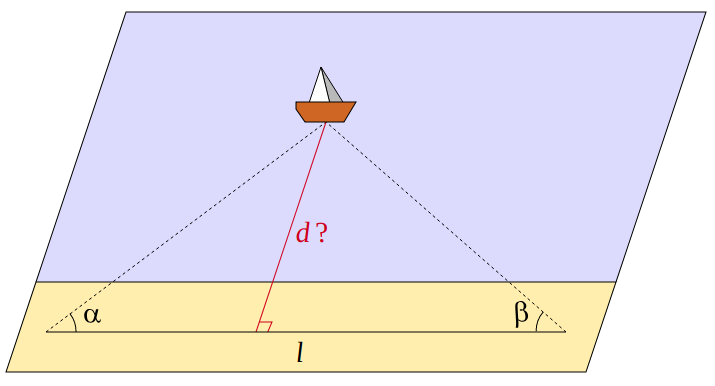This imposes restrictions on how to compute distances in some interesting geometrical instances. For example, we could redefine the concept of height of a triangle to be simply the distance from one vertex to the opposing side of the triangle. In this case, the triangle area gets also redefined in terms of distance, since the area is a function of the height of the triangle.

## Distance to a line and between 2 lines

Let's look at couple examples in 2D space. To calculate the distance between a point and a straight line we could go (calculate the segment perpendicular to the line from the line to the point and the compute its length) or we could simply : d = |Ax1 + By1 + C | / √(A2 + B2) where the line is given by Ax+By+C = 0 and the point is defined by (x1, y1).

The only problem here is that a straight line is generally given as y = mx + b so we would need to convert this equation to the previously show form: y = mx + b → mx - y + b = 0 so we can see that  A = m,  B = -1  and  C = b. This leaves the previous equation with the following values: d = |mx1 -y1 + b | / √(m2 + 1).

For the distance between 2 lines, we just need to compute the length of the segment that goes from one to the other and is perpendicular to both. Once again, there is a : d= |C2-C1|/√(A2+B2) if the lines are A1 x+B1 y+C1 = 0 and A2x+B2y+C2 = 0. We can also convert to slope intercept for and obtain: d= |b2-b1|/√(m2+1) for lines y = m1x + b1 and y = m2x + b2.

Notice that both line needs to be parallel since otherwise the would touch at some point and their distance would then be  d = 0. That's the reason the formulas omit most of the subscripts since for parallel lines: A1 = A2 = A and B1 = B2 = B while in slope intercept form parallel lines are those for which m1 = m2 = m

Apart from perpendicularity, another important concept to talk about regarding distance, is the midpoint. This is the point that is precisely in the middle between the two others. The midpoint is defined as the point that is the same distance away from each of the points of reference. We can and will generalize this concept in a later section, but for now, we can limit ourselves to geometry. For example, the midpoint of any diameter in a circle or even a sphere is always the centre of said object.

## How to find the distance using our distance calculator

As we have mentioned before, distance can mean many things, which is why we have provided a few different options for you in this calculator. You can calculate the distance between a point and a straight line, the distance between two straight lines (they always have to be parallel), or the distance between points in space. When it comes to calculating the distances between two point, you have the option of doing so in 1, 2, 3, or 4 dimensions. I know, I know, 4 dimensions sounds scary, but you don't need to use that option. And you can always learn more about it by reading and playing around with the calculator. We promise it won't break the Internet or the universe.

We have also added the possibility for you to define 3 different points in space, from which you will obtain the 3 pairs of distances between them, so, if you have more than two points, this will save you time. The number of dimensions you are working in will determine the number of coordinates that describe a point, which is why, as you increase the number of dimensions, the calculator will ask for more input values.

Even though using the calculator is very straightforward, we still decided to include a step-by-step solution. This way you can get acquainted with the distance formula and how to use it (as if this was the 1950's and the Internet was still not a thing). Now let's take a look at a practical example: How to find the distance between two points in 2-D.

Suppose you have two coordinates, (3, 5) and (9, 15), and you want to calculate the distance between them. To calculate the 2-D distance between these two points, follow these steps:

1. Input the values into the formula: √[(x₂ - x₁)² + (y₂ - y₁)²].
2. Subtract the values in the parentheses.
3. Square both quantities in the parentheses.
5. Take the square root.
6. Use the distance calculator to check your results.

Working out the example by hand, you get:

√[(9 - 3)² + (15 - 5)²] = √[(6)² + (10)²] = √[36 + 100] = √136,

which is equal to approximately 11.66. Note, that when you take the square root, you will get a positive and negative result, but since you are dealing with distance, you are only concerned with the positive result. The calculator will go through this calculations step by step to give you the result in exact and approximate formats.

## Driving distance between cities: a real-world example

Let's take a look of one of the applications of the distance calculator. You can use it together with the gas calculator for making road trip plans. Suppose you are traveling between cities A and B, and the only stop is in city C, with a route A to B perpendicular to route B to C. We can determine the distance from A to B, and then, with the gas calculator, determine fuel cost, fuel used and cost per person while traveling.

The difficulty here is to calculate the distances between cities accurately. A straight line (like what we use in this calculator) can be a good approximation, but it can be quite off if the route you're taking is not direct but takes some detour, maybe to avoid mountains or to pass by another city. In that case, just use Google maps or any other tool that calculates the distance along a path not just the distance from one point to another as the crow flies.Where our calculator can give proper measurements and predictions, is when calculating distances between objects, not the length of a path. With this in mind, there are still multiple scenarios in which you might actually be interested in the distance between objects, regardless of the path you would have to take. One such example is the distance between astronomical objects.

## Distance from Earth to Moon and Sun - astronomical distances

When we look at a distance within our Earth, it is hard to go far without bumping into some problems, from the intrinsic curvature of this space (due to the Earth curvature being non-zero) to the limited maximum distance between two points on the Earth. It is because of this, and also because there is a whole universe beyond our Earth, that distances in the universe are of big interest for many people. Since we have no proper means of interplanetary traveling, let alone interstellar travels, let's focus for now on the actual Euclidean distance to some celestial objects. For example the distance from the Earth to the Sun, or the distance from the Earth to the Moon.

These distances are beyond imaginable for our ape-like brains. We struggle to comprehend the size of our planet, never mind the vast, infinite universe. This is so difficult that we need to use either scientific notation or light years, as a unit of distance for such long lengths. The longest trips you can do on Earth are barely a couple thousand kilometers, while the distance from Earth to the Moon, the closest astronomical object to us, is 384,000 km. On top of that, the distance to our closest star, that is the distance from Earth to the Sun, is 150,000,000 km or a little over 8 light minutes.When you compare these distances with the distance to our second nearest star (Alpha Centauri), which is 4 light years, suddenly they start to look much smaller. If we want to go even more ridiculous in comparison we can always think about a flight from New York to Sydney, which typically takes more than 20 h and it's merely over 16,000 km, and compare it with the size of the observable universe, which is about 46,600,000,000 light years!

Here, we have inadvertently risen a fascinating point, which is that we measure distances not in length but in time. Thus, we extend the notion of distance beyond its geometrical sense. We will explore this possibility in the next section as we speak about the importance and usefulness of distance beyond the purely geometrical sense. This is a very interesting path to take and is mostly inspired by the philosophical need to extend every concept to have a universal meaning, as well as from the obvious physical theory to mention, when talking about permutations of the space and time, or any other variable that can be measured.

## Distance beyond length

Typically, the concept of distance refers to the geometric Euclidean distance and is linked to length. However, you can extend the definition of distance to mean just the difference between two things, and then a world of possibilities opens up. Suddenly one can decide what is the best way to measure the distance between two things and put it in terms of the most useful quantity. A very simple step to take is to think about the distance between two numbers, which is nothing more than the 1D difference between these numbers. To obtain it, we simply subtract one from the other and the result would be the difference, a.k.a. the distance.

We could jump from this numerical distance to, for example, difference or distance in terms of the percentage difference, which in some cases might provide a better way of comparison. We don't need to stay just with percentage, we can convert percentage to fraction if you feel that would be the best way to express distance. But so far, this is still just one level of abstraction in which we simply remove the units of measurement. But what if we were to use different units altogether?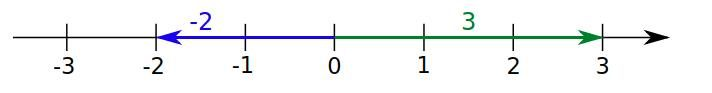By extending the concept of distance to mean something closer to difference, we can calculate the difference between two temperatures in terms of degrees or thermal energy, or other related quantity like pressure. But we don't need to get really extreme, let's see how two points can be separated by a different distance, depending on the assumptions made. Coming back to the driving distance example, we could measure the distance of the journey in time, instead of length. In this case, we need an assumption to allow such translation; namely the way of transport.

There is a big difference in the time taken to travel 10 km by plane versus the time it takes by car; or by car versus bike. Sometimes, however, the assumption is clear and implicitly agreed on, like when we measure the lightning distance in time which we then convert to length. This brings up an interesting point, that the conversion factor between distances in time and length is what we call speed or velocity (remember they are not exactly the same thing). Truth be told, this speed doesn't have to be constant as exemplified by accelerated motions such as that of a free fall under gravitational force, or the one that links stopping time and stopping distance via the breaking force and drag or, in very extreme cases, via the force of a car crash.Another place where you can find weird units of distance are in solid state physics, where the distance a particle travels inside of a material is often expressed as an average of interactions or collisions. This distance is linked to length by using the mean free path which is the mean distance (in length) a particle travels between interactions. If we want to get even more exotic we can think about the distance from the present value to the future value of something like a car. This distance between prices is linked here by the car depreciation, and it's not as cut and dry as the other distances, but only because of the number of factors involved in calculating this distance.

We don't want to, however, make anyone's brain explode, so please don't think too hard about this. Just take this calculator and use it for length-based distance in 2D space. You can always return to this philosophical view on distances if you ever find yourself bored and having already checked all of our .

## FAQ

### How to find the distance between two points?

To find the distance between two points we will use the distance formula: √[(x₂ - x₁)² + (y₂ - y₁)²]

1. Get the coordinates of both points in space
2. Subtract the x-coordinates of one point from the other, same for the y components
3. Square both results separately
4. Sum the values you got in the previous step
5. Find the square root of the result above.

If you think this is too much effort you can simply use the Distance Calculator from Omni

### Is distance a vector?

Distance is not a vector. The distance between points is a scalar quantity, meaning it is only defined by its value. However, the displacement is a vector with value and direction. So the distance between A and B is the same as the distance from B to A, but the displacement is different depending on their order.

### What is a click in distance?

Click is slang for a kilometer which is 0.62 miles. It is actually written with "k" (Klick) as it is derived from the word kilometer. It is commonly used in the military and motorcyclists.

### What is the distance formula?

The distance formula is: √[(x₂ - x₁)² + (y₂ - y₁)²]. This works for any two points in 2D space with coordinates (x₁, y₁) for the first point and (x₂, y₂) for the second point. You can memorize it easily if you notice that it is Pythagoras theorem and the distance is the hypothenuse, and the lengths of the catheti are the difference between the x and y components of the points.

### How to find the distance of a vector?

The distance of a vector is its magnitude. If you know its components:

1. Take each of the components of the vector and square them
2. Sum them up
3. Find the square root of the previous result
4. Enjoy the good work!

If you know its polar representation, it will be a number and an angle. That number is the magnitude of the vector, which is its distance.

### What is the SI unit of distance?

The SI unit of distance is the meter, abbreviated to "m". A meter is approximately 3.28 feet. Other common units in the International System of units are the centimeter (one one-hundredth of a meter, or 0.39 inches) and the kilometer (one thousand meters or 0.62 miles), among others.

### What is the distance from a to b?

The distance from A to B is the length of the straight line going from A to B. The distance from B to A is the same as the distance from A to B because distance is a scalar

### What is the dimension of distance?

Distance is a measure of one-dimensional space. The distance between two points is the shortest length of 1D space between them. If you divide distance over time you will get speed, which has dimensions of space over time.

### Is light-year time or distance?

A light-year is a measurement of distance. It is 9.461⨉1012 kilometers or 5.879⨉1012, which is the distance traveled by a ray of light in a perfect vacuum over the span of a year.

### How to solve for distance with velocity and time?

The velocity and the moving time of an object you can calculate the distance:

1. Make sure the speed and time have compatible units (miles per hour and hours, meter per second, and seconds...)
2. If they aren't, convert them to the necessary units
3. Multiply the velocity by the time
4. The result should be the distance traveled in whichever length units your speed was using!
Álvaro Díez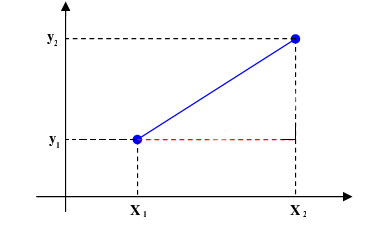Type
2 points
Dimensions
2D
First point
x₁
y₁
Second point
x₂
y₂
Result
Distance
People also viewed…

### Chilled drink

With the chilled drink calculator you can quickly check how long you need to keep your drink in the fridge or another cold place to have it at its optimal temperature. You can follow how the temperature changes with time with our interactive graph.

### Grams to cups

The grams to cups calculator converts between cups and grams. You can choose between 20 different popular kitchen ingredients or directly type in the product density.

### Involute function

Calculate the involute function in a single easy step using our involute function calculator.

### Sum and difference identities

The sum and difference identities calculator is here to help you whenever you need to find the trigonometric function (all six of them!) of a sum or difference of two angles.